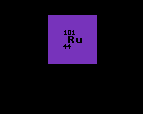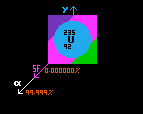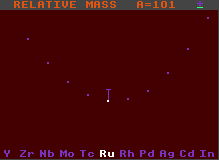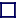Stable against alpha decayUnstable to alpha decay

### ALPHA STABILITY

RELATIVE MASS (ALPHA STABILITY)
There is a line in the centre of the chart corresponding to alpha stability. The length of the line is proportional to the difference in atomic mass between the parent nucleus and the daughter product plus an alpha particle. If the parent nucleus is the heavier, then it can decay by alpha decay and is shown as a downward green line, but if the parent nucleus is the lighter, then the nucleus cannot decay by alpha emission, and is shown as an upward blue line. The longer the green line, the greater is the likelihood of decay by alpha emission and the shorter the halflife. The difference in atomic mass in MeV is to the same scale as that for beta and inverse beta decay, and is shown above in green numbers. This is the decay energy, the particle energies are always lower.

Generally, the longer the green line, the greater is the kinetic energy imparted to the emitted alpha particle, although some of this energy is taken up by recoil velocity of the daughter product. (Note that the end of the green line does not represent the daughter product, that is two elements to the left hand side and four units of atomic weight, A, less, and hence cannot be properly represented on the same chart).

Note also that not every nuclide which is unstable to alpha decay is declared as such on the colour coded Segre chart, probably because the alpha decay is either extremely infrequent (i.e. of very long halflife) or the branching ratio for the alpha decay route is very much less than that for the other route(s) shown.RELATIVE MASS of A=101 ISOBARS
Fig 1 shows ruthenium-101 which is stable to alpha decay.RELATIVE MASS of A=235 ISOBARS
Fig 2 shows uranium-235 which is unstable to alpha decay liberating 4.67Mev.

Both the above examples are stable to beta/inverse beta decay, but this is not a requirement for alpha decay, it just made the diagram clearer to chose one stable to beta/inverse beta decay.